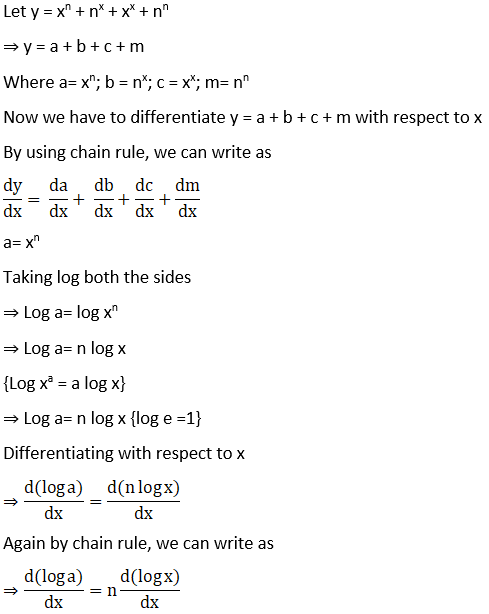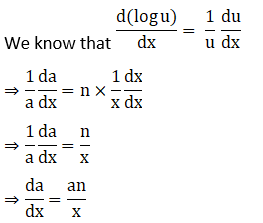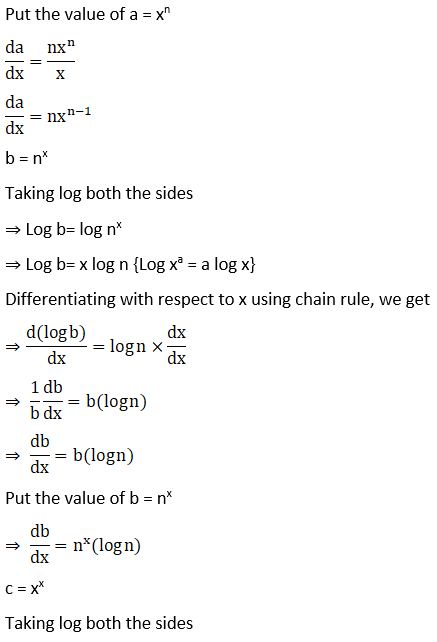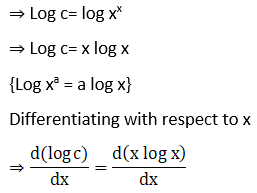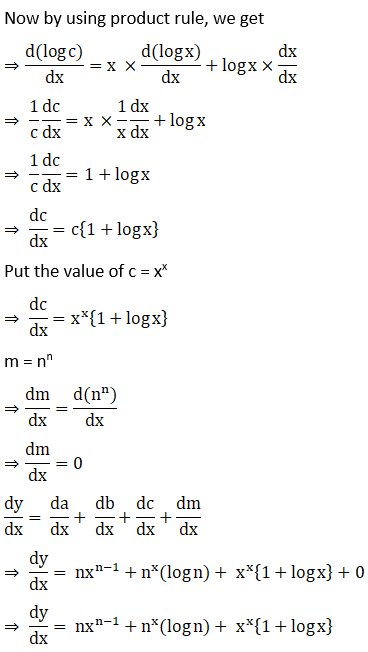# RD Sharma Solutions for Class 12 Maths Exercise 11.5 Chapter 11 Differentiation

RD Sharma Solutions for Class 12 Maths Exercise 11.5 Chapter 11 Differentiation is provided here. Logarithmic differentiation is the main topic covered in this exercise. The logarithmic function is an inverse of an exponential function. This exercise explains how to differentiate the given logarithmic function and algorithm, including logarithmic differentiation.

The set of experts at BYJU’S conducts vast research on each topic before designing the solutions as per the prescribed syllabus of CBSE. To know more about these topics, students can refer to RD Sharma Solutions for Class 12 Maths Exercise 11.5 of Chapter 11 Differentiation.

## RD Sharma Solutions for Class 12 Differentiation Exercise 11.5

### Access other exercises of RD Sharma Solutions for Class 12 Chapter 11 – Differentiation

Exercise 11.1 Solutions

Exercise 11.2 Solutions

Exercise 11.3 Solutions

Exercise 11.4 Solutions

Exercise 11.6 Solutions

Exercise 11.7 Solutions

Exercise 11.8 Solutions

### Access answers to Maths RD Sharma Solutions for Class 12 Chapter 11 – Differentiation Exercise 11.5

Exercise 11.5 Page No: 11.88

Differentiate the following functions with respect to x:

1. x1/x

Solution: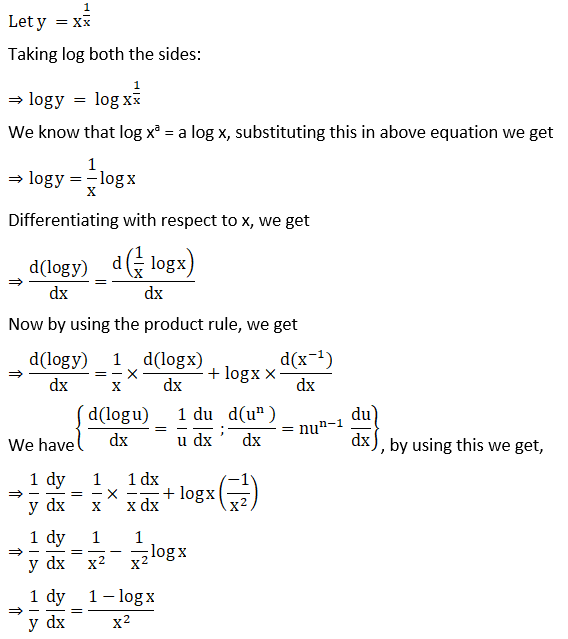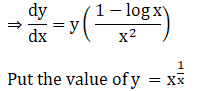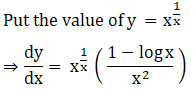2. xsin x

Solution: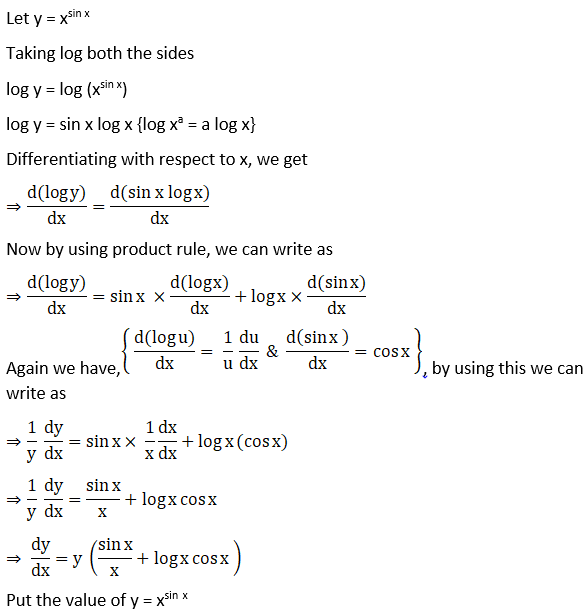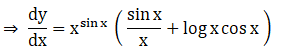3. (1 + cos x)x

Solution: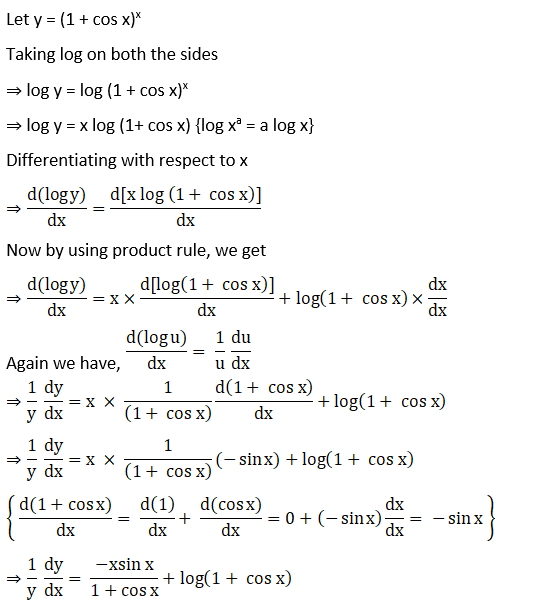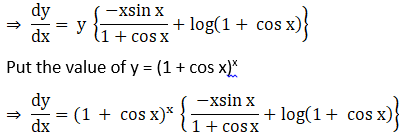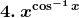Solution: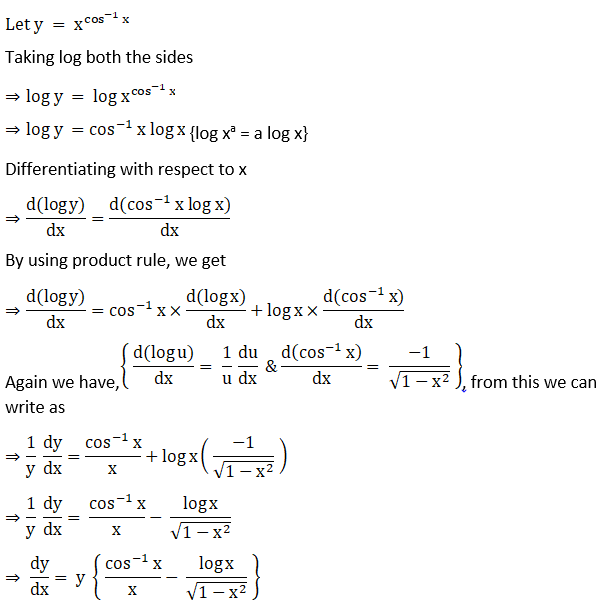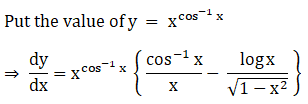5. (log x)x

Solution: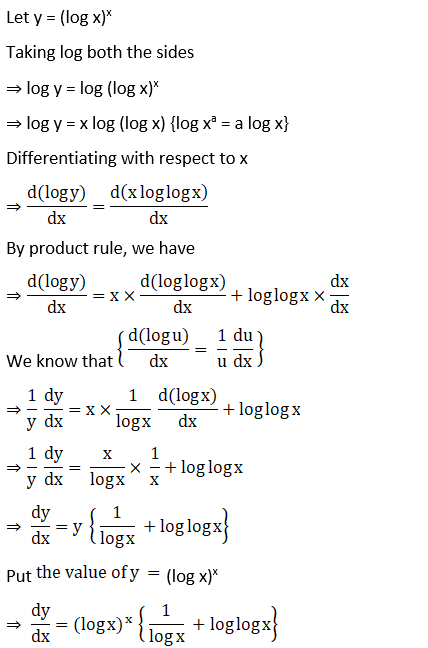6. (log x)cos x

Solution:

Let y = (log x)cos x

Taking log both sides, we get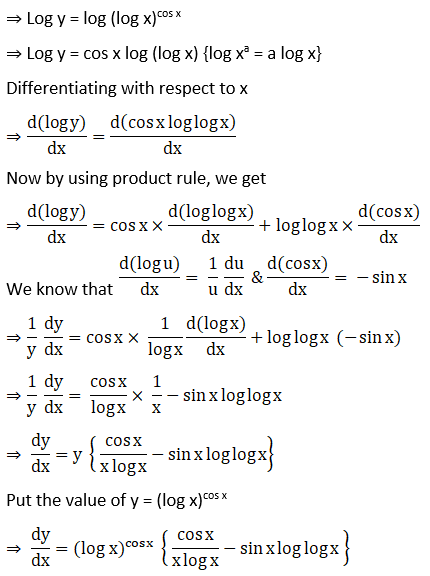7. (Sin x)cos x

Solution: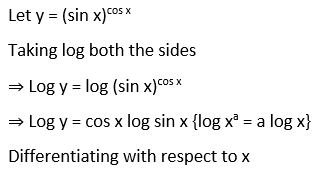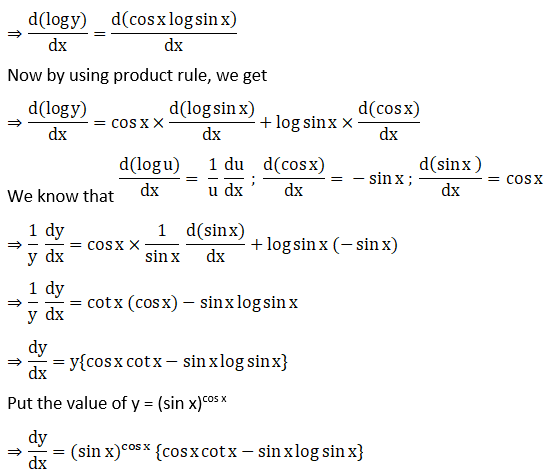8. ex log x

Solution: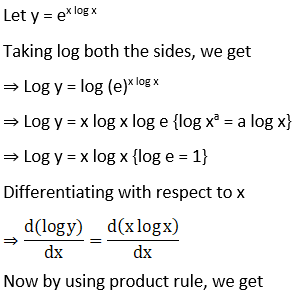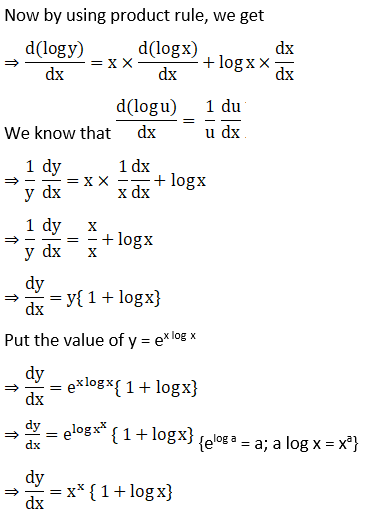9. (Sin x)log x

Solution: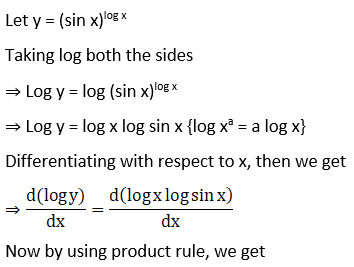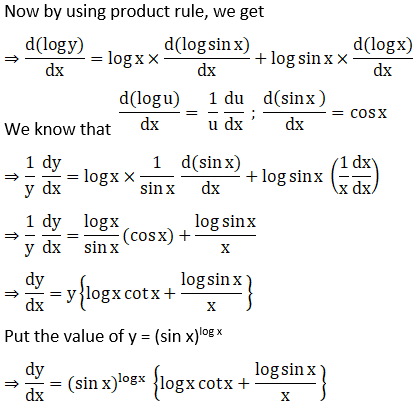10. 10log sin x

Solution: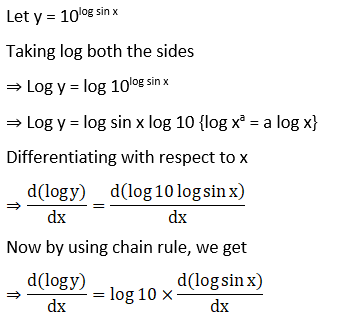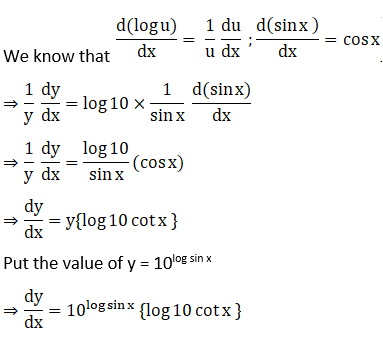11. (log x)log x

Solution: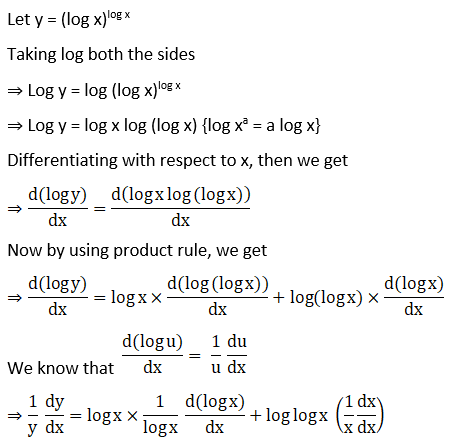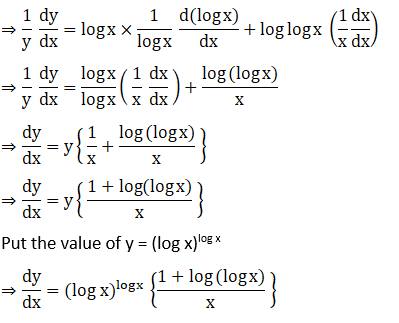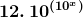Solution: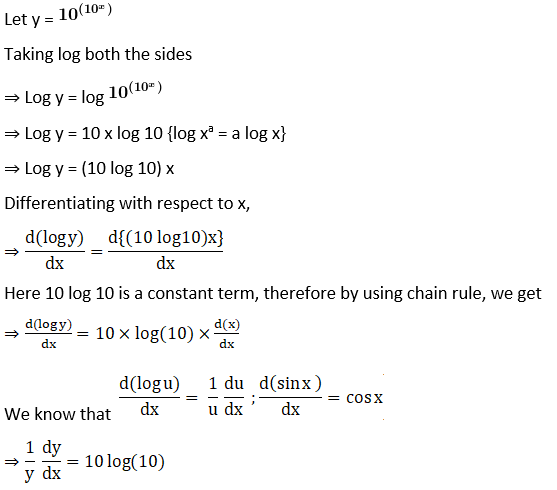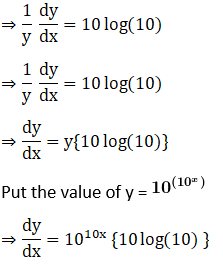13. Sin (xx)

Solution: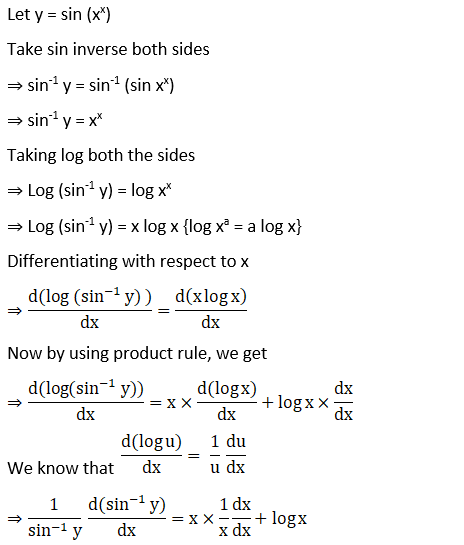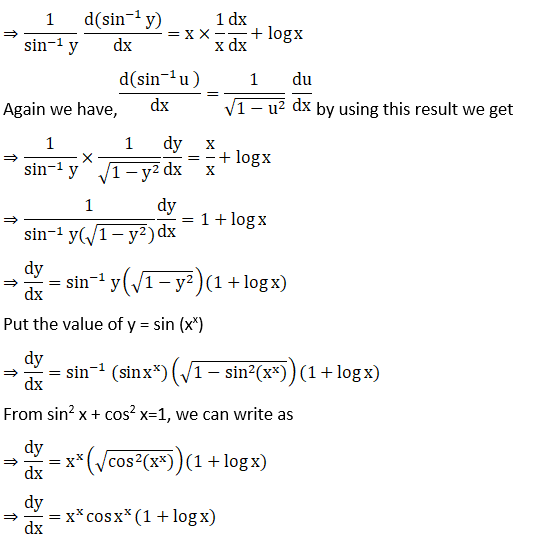14. (Sin-1 x)x

Solution: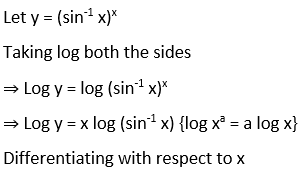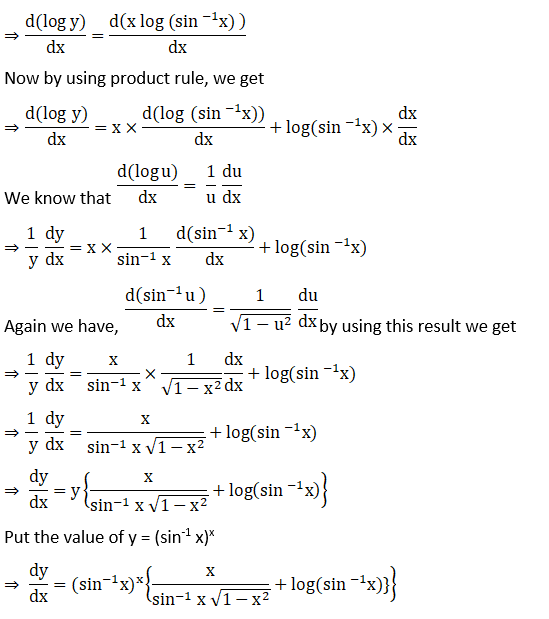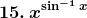Solution: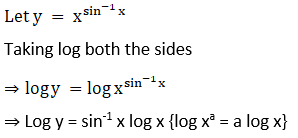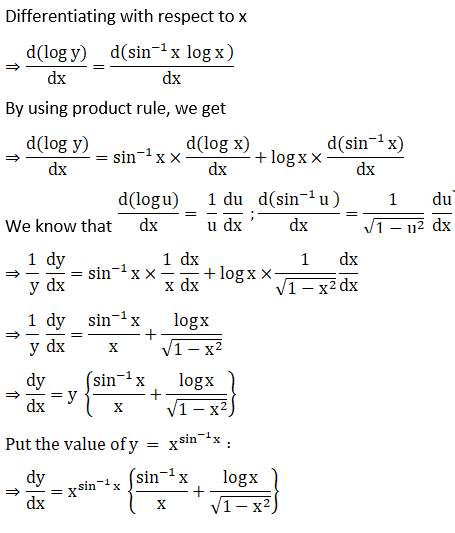16. (tan x)1/x

Solution: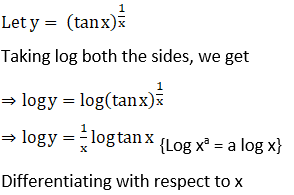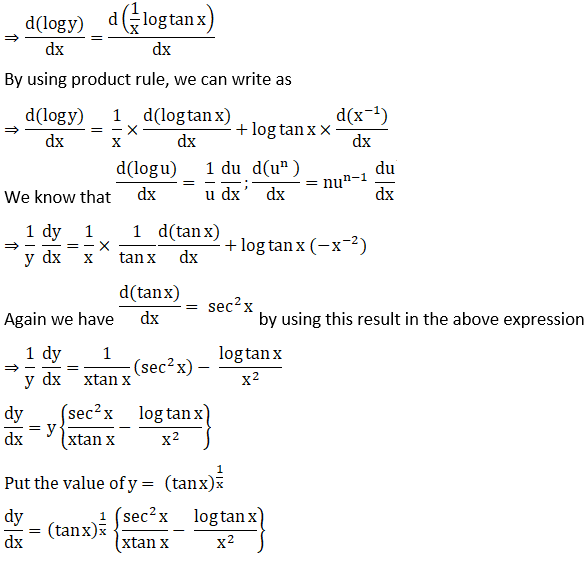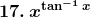Solution: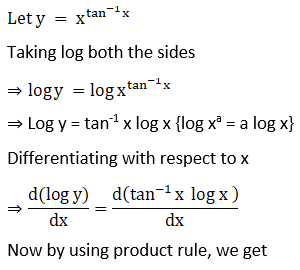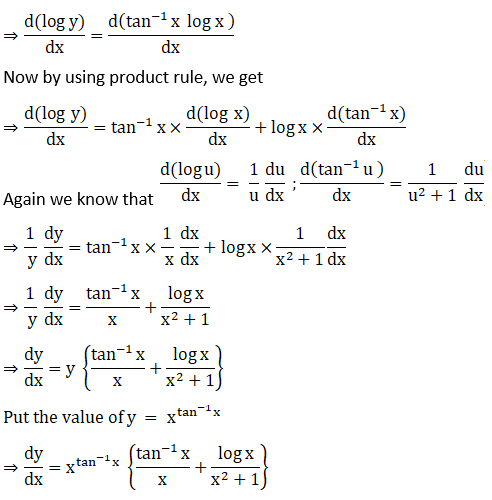18. (i) (xx) √x

Solution: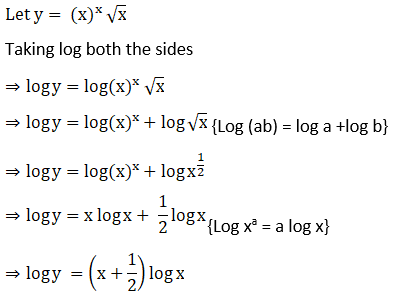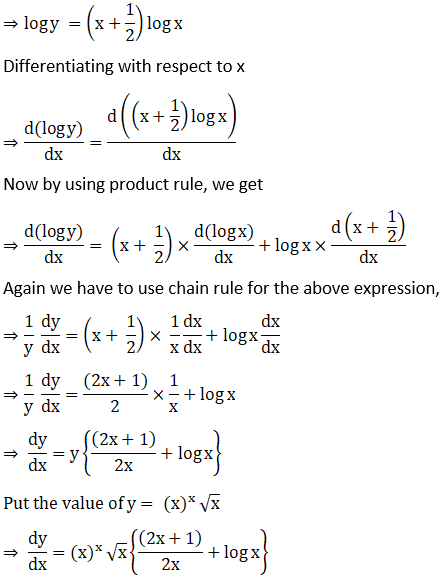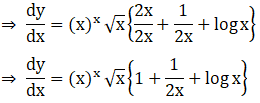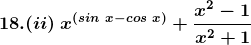Solution: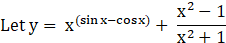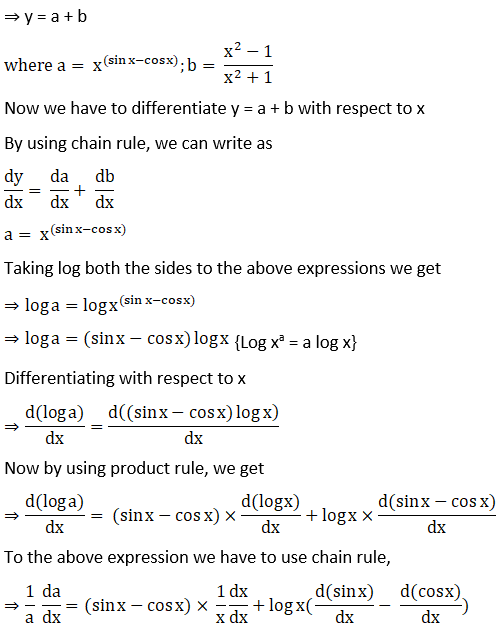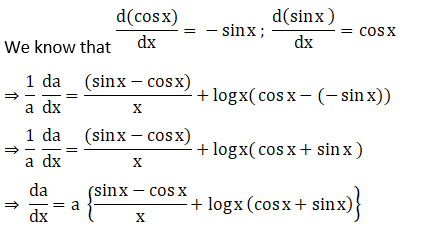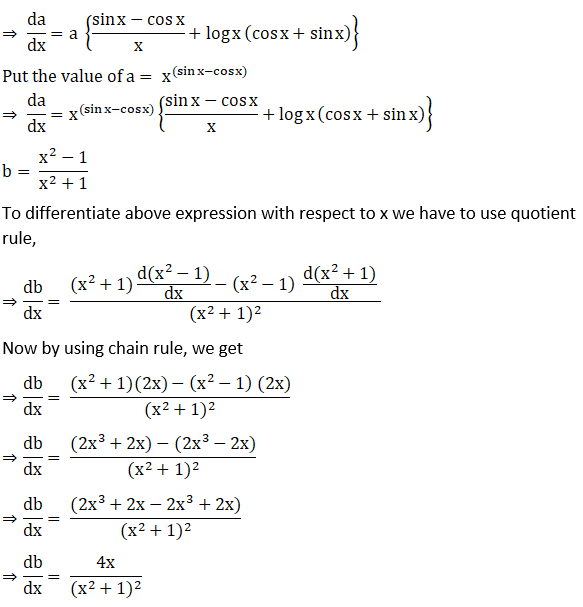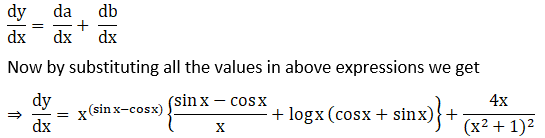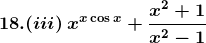Solution: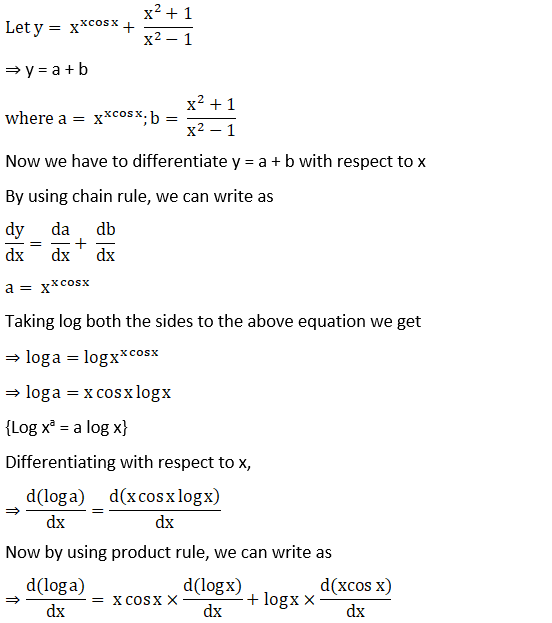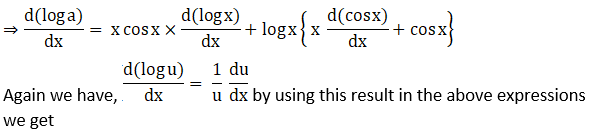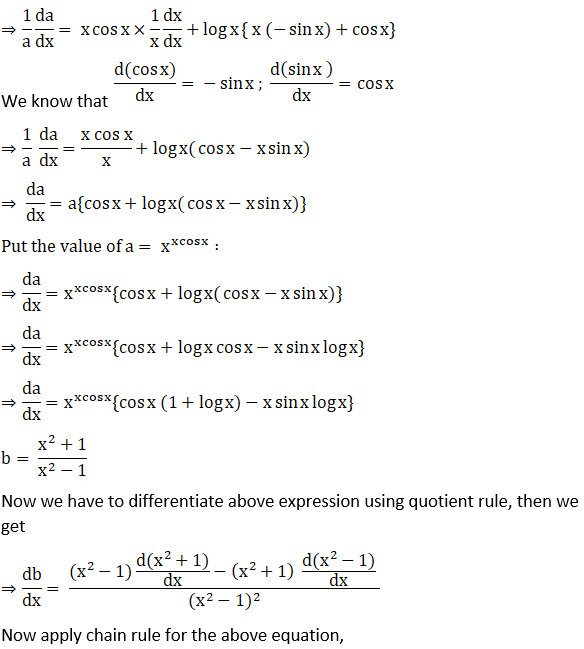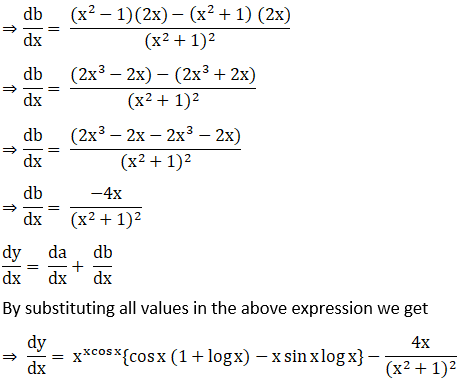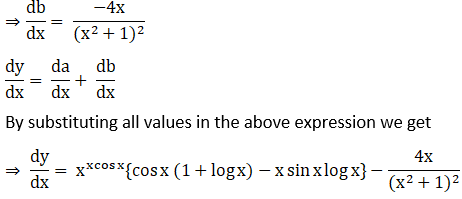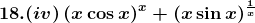Solution: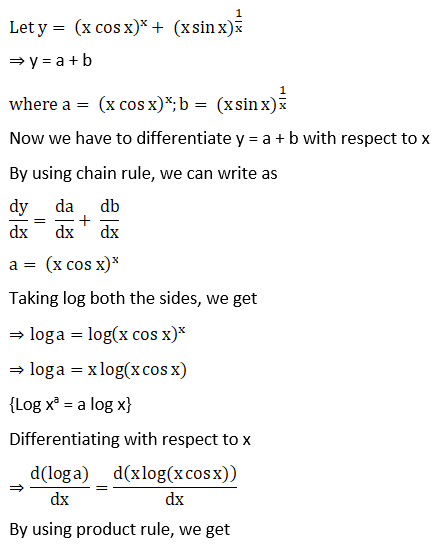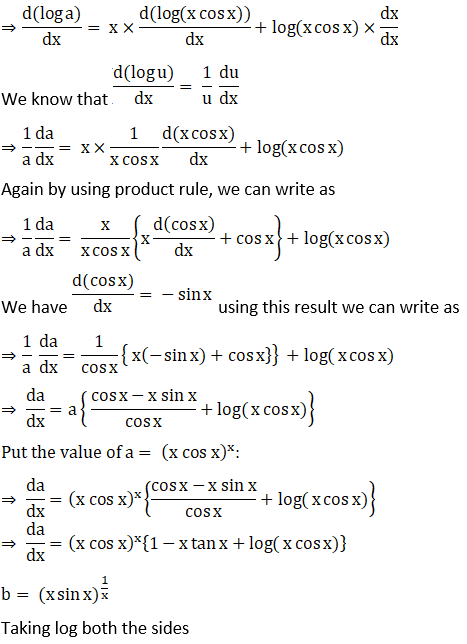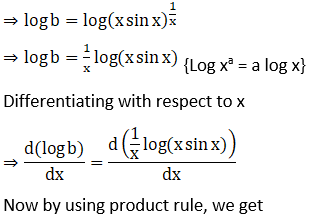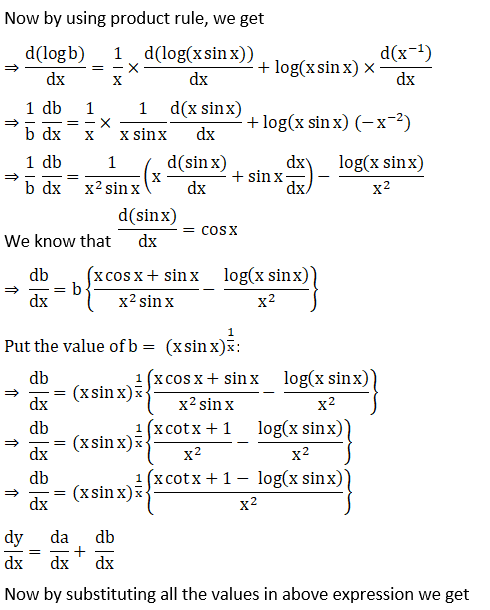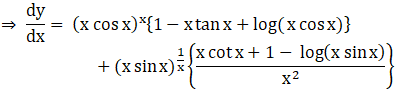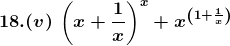Solution: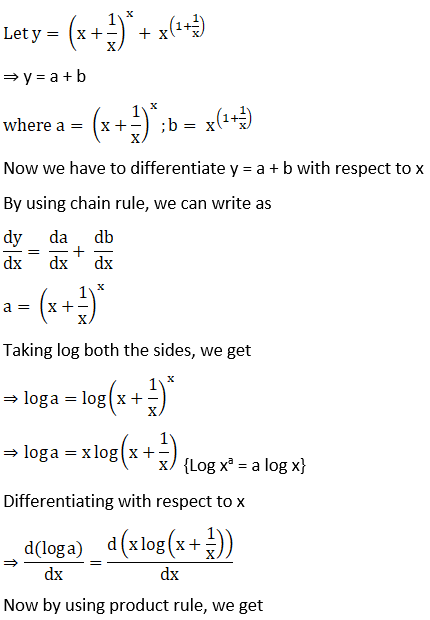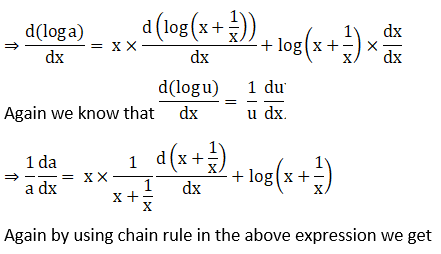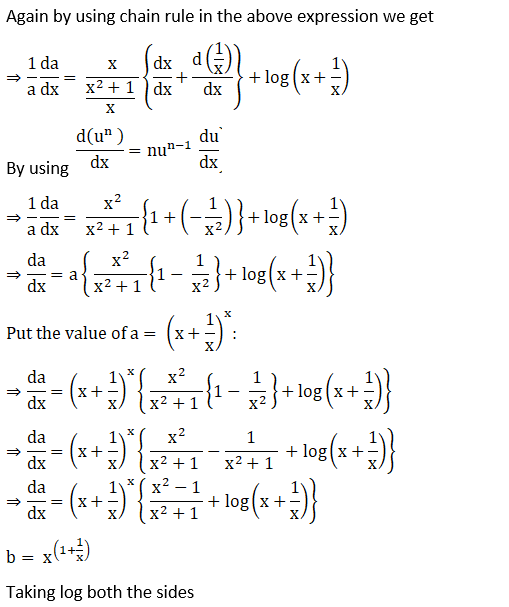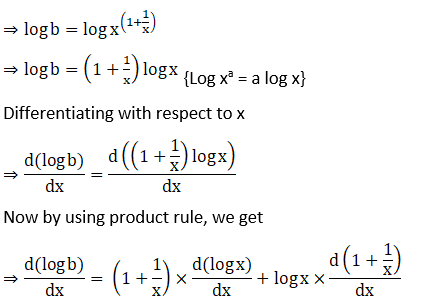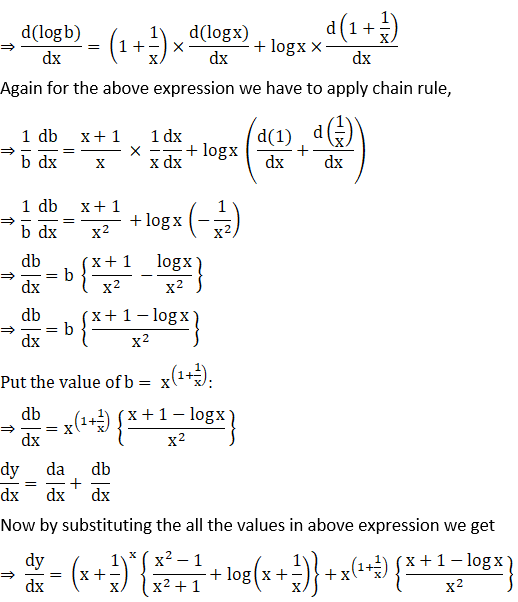18. (vi) esin x + (tan x)x

Solution: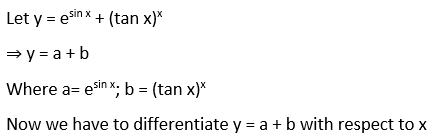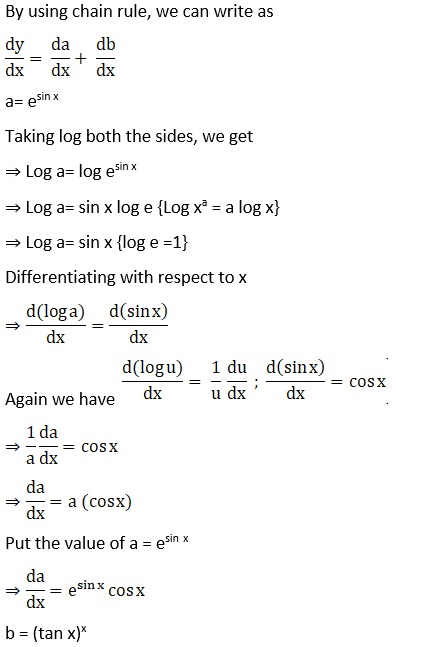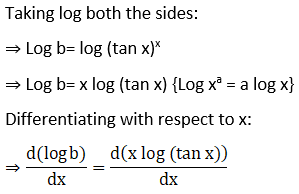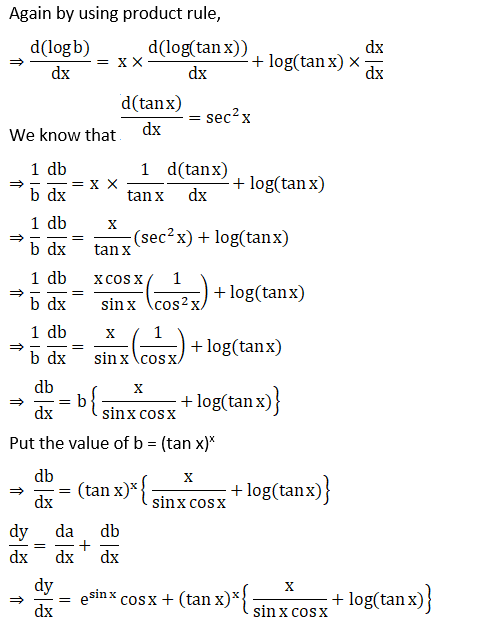18. (vii) (cos x)x + (sin x)1/x

Solution: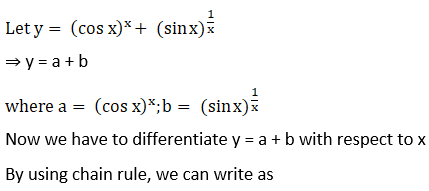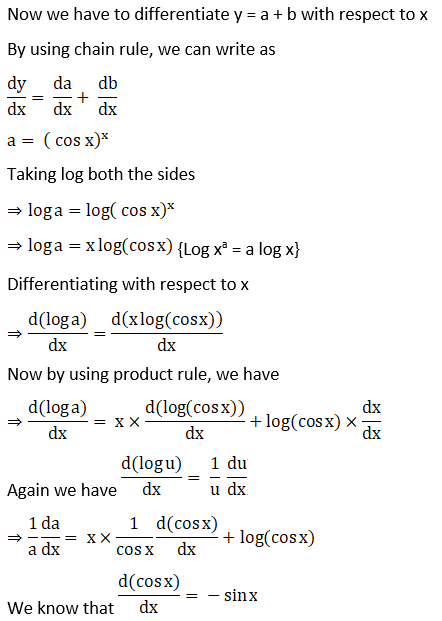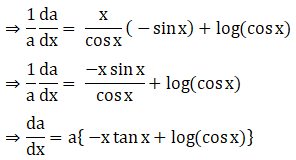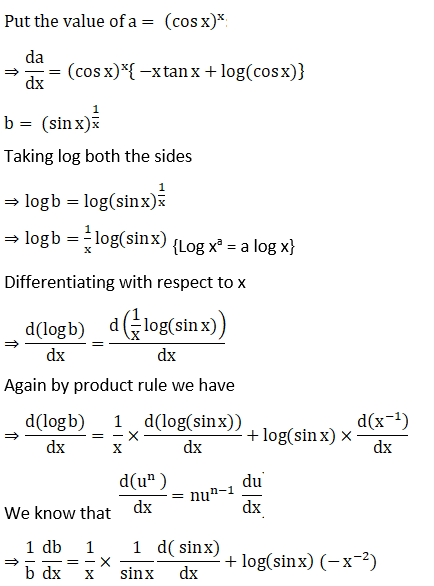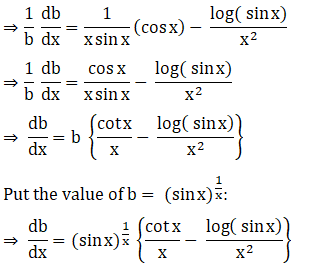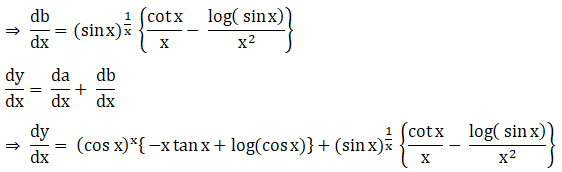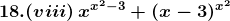Solution: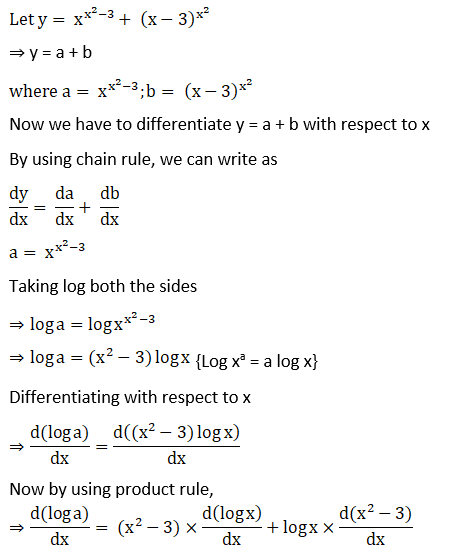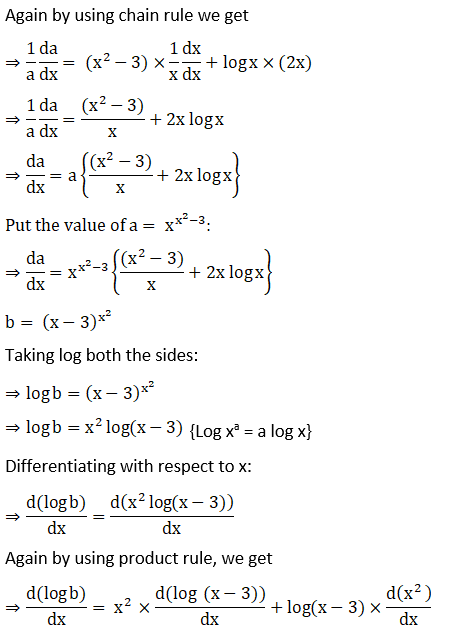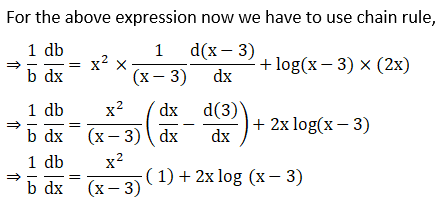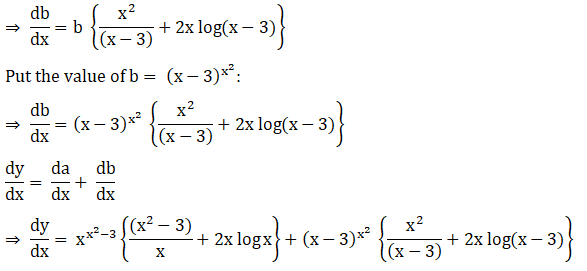19. y = ex + 10x + xx

Solution: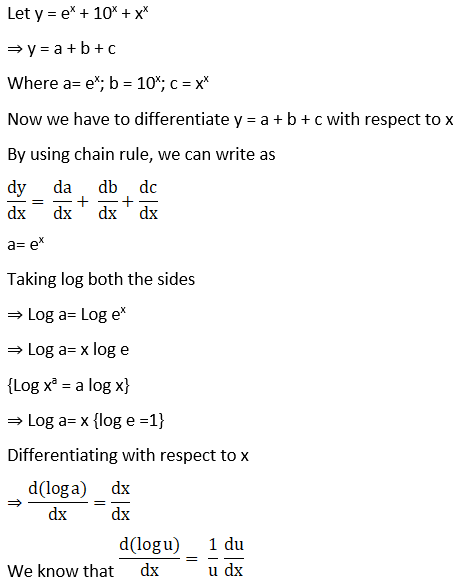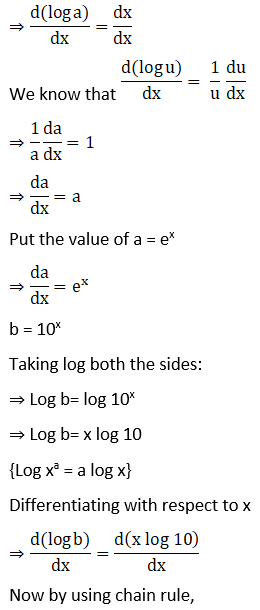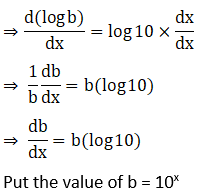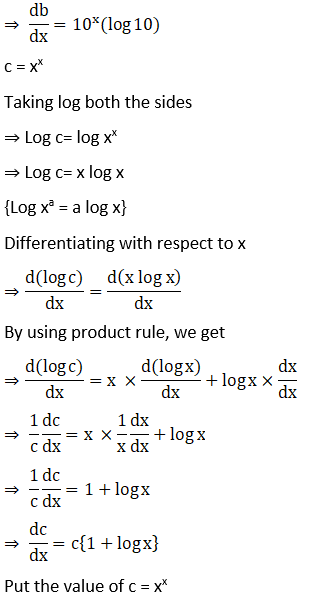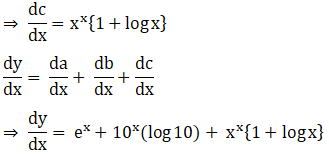20. y = xn + nx + xx + nn

Solution: